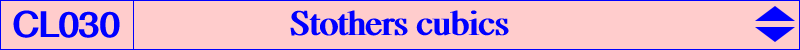Contributed by Wilson Stothers (private message 2004-05-10) with some additions by myself A Stothers cubic is a cubic of the form ST(F) = cK0(#F,TG(F)), where TG(F) denotes the tripolar centroid of F (see a definition at CL029). These cubics are always of type cK0 since TG(F) lies on the tripolar of F. These have all the properties of a cK0, but are unusual since we can identify the nodal tangents. Notations For any P, T(P) is the tripolar of P, P* is the isoconjugate of P in the isoconjugation with F as fixed point, C(P) is the circumconic with perspector P, I(P) is the inconic with perspector P. *** General properties of cK0(#F,R) - so that the root R is on T(F). T(R*) is also the tangent to C(R) at F and the polar of R with respect to C(F). R* lies on C(F) cK0(#F,R) is the locus of X such that XX* is divided harmonically by the lines T(F) and T(R*). cK0(#F,R) is the F-Hirst inverse of C(R). the nodal tangents of cK0(#F,R) are the tripolars of the intersections of T(R*) and C(F). the nodal tangents of cK0(#F,R) are the tangents from F to I(R*). the pivotal conic of cK0(#F,R) is the dual of C(R) with respect to C(F). cK0(#F,R) includes : F, F1 the fourth intersection of C(F) and C(R) i.e. the trilinear pole of the line FR, F2 the intersection of T(F) and T(R*). Notice that F1 and F2 are isoconjugate points on the cubic. R*, F and F1 are collinear - so F1, R* are F-antipodes on C(F). The pivotal conic and I(R*) touch at R, with common tangent T(F). For example, with F = I (incenter), we obtain isogonal cubics and T(F) is the antiorthic axis. In particular, when R = X(650), X(513), X(661) we find K040 (the Pelletier strophoid), K137 = Z+(IK), K221 respectively. *** The special case ST(F) = cK0(#F,TG(F)). Say F has barycentrics (f : g : h). Observe that the intersections in property 3 are the points P1, P2 with first barycentrics f/(g-h) and f/(g+h-2f). This gives the nodal tangents as T1 = T(P1), the line through F and F^2 and the G-Ceva conjugate of F ( = centre of C(F)). T2 = T(P2), the line through F and the infinite point of T(F). If F is at infinity (class CL029) then T2 is the line at infinity. Otherwise, T2 is the line through F parallel to T(F). The pivotal conic is the inconic of the anticevian triangle of F touching (any two of) T(F), T1, T2. The general equation of ST(F) is : f(g - h)(g + h - 2f)x(h^2y^2 + g^2z^2) + cyclic = 0. Example : ST(K) with K = X(6). Then TG(K) = X(351), the centre of the Parry Circle. C(K) is the Circumcircle. C(R) includes K, X(187), X(249), X(512), X(524), X(598), X(843) T(K) is the Lemoine Axis. T(R*) is the line through K, X(110) and X(111). R* = X(691). F1 = X(843). The points P1, P2 above are X(110), X(111) (on the Parry Circle and the circumcircle). T1 is the Brocard Axis T2 is the line through K parallel to the Lemoine Axis. ST(K) includes K and X(843). The other K-Hirst inverses are unlisted. The pivotal conic is the inconic of the tangential triangle touching the Brocard and Lemoine Axes (as well as T2). Observe that T1 and T2 are perpendicular. This is true for any ST(F) with F on the quintic Q012. We find several other interesting cubics with the same singularity K such as K222, K223, K224, K225 with roots X(512), X(647), X(649), X(663) respectively.